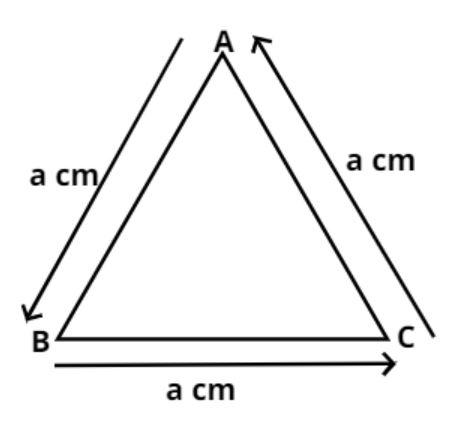QuestionAnswers

# If the area of an equilateral triangle is $36\sqrt 3 {\text{ c}}{{\text{m}}^2}$, find its perimeter.Verified
114K+ Views
Hint: Let the side of equilateral triangle be a cm, use the direct formula of area of equilateral triangle which is $\dfrac{{\sqrt 3 }}{4}{a^2}$, to get the value of a. Then use the concept that perimeter is defined as the sum of all sides of the triangle.As we know that the area of the equilateral triangle is $\dfrac{{\sqrt 3 }}{4}{a^2}$ square unit where (a) is the side of the equilateral triangle.
And we also know that the perimeter of any shape is the sum of all the sides.
And we know that in an equilateral triangle all the sides are equal as shown in figure.
So the perimeter (S) of the equilateral triangle is
$\Rightarrow S = a + a + a = 3a$................. (1)
Now it is given that the area of the equilateral triangle is $36\sqrt 3 {\text{ c}}{{\text{m}}^2}$.
So equate this value to the formula of the area of the equilateral triangle and calculate the length of the side.
$\Rightarrow \dfrac{{\sqrt 3 }}{4}{a^2} = 36\sqrt 3$
Now simplify this equation we have,
$\Rightarrow \dfrac{1}{4}{a^2} = 36$
$\Rightarrow {a^2} = 36 \times 4 = 144 = {\left( {12} \right)^2}$
$\Rightarrow a = 12$ cm
So the side of the equilateral triangle is 12 cm.
Now calculate the perimeter of the equilateral triangle according to equation (1) we have,
$\Rightarrow S = 3a = 3 \times 12 = 36$ cm.
So the perimeter of the equilateral triangle is 36 cm.
So this is the required answer.

Note: An equilateral triangle is one whose all three sides are equal, this fact along with its property that all the angles of an equilateral triangle are ${60^0}$, differs it from other triangles like isosceles triangles in which only two sides are equal. It is advised to grasp the direct formula of the area of the equilateral triangle.